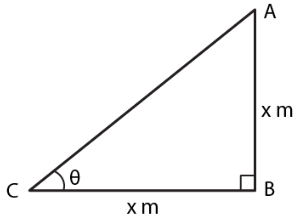Guru

# What is the angle of elevation of the sun when the length of the shadow of a vertical pole is equal to its height.

• 0

sir this is the question from the book -ML aggarwal( avichal publication) class 10th , chapter20 , heights and distances., it is an important question of this chapter
we have to find the  angle of elevation of the sun when the length of the shadow of a vertical pole is equal to its height. explain in detail
question no 4 , heights and distances , ICSE board

Share

1. Consider AB as the pole and CB as its shadow

θ is the angle of elevation of the sun

Take AB = x m and BC = x m

We know that

tan θ = AB/CB = x/x = 1

So we get

tan 450 = 1

θ = 450Hence, the angle of elevation of the sun is 450.

• 0